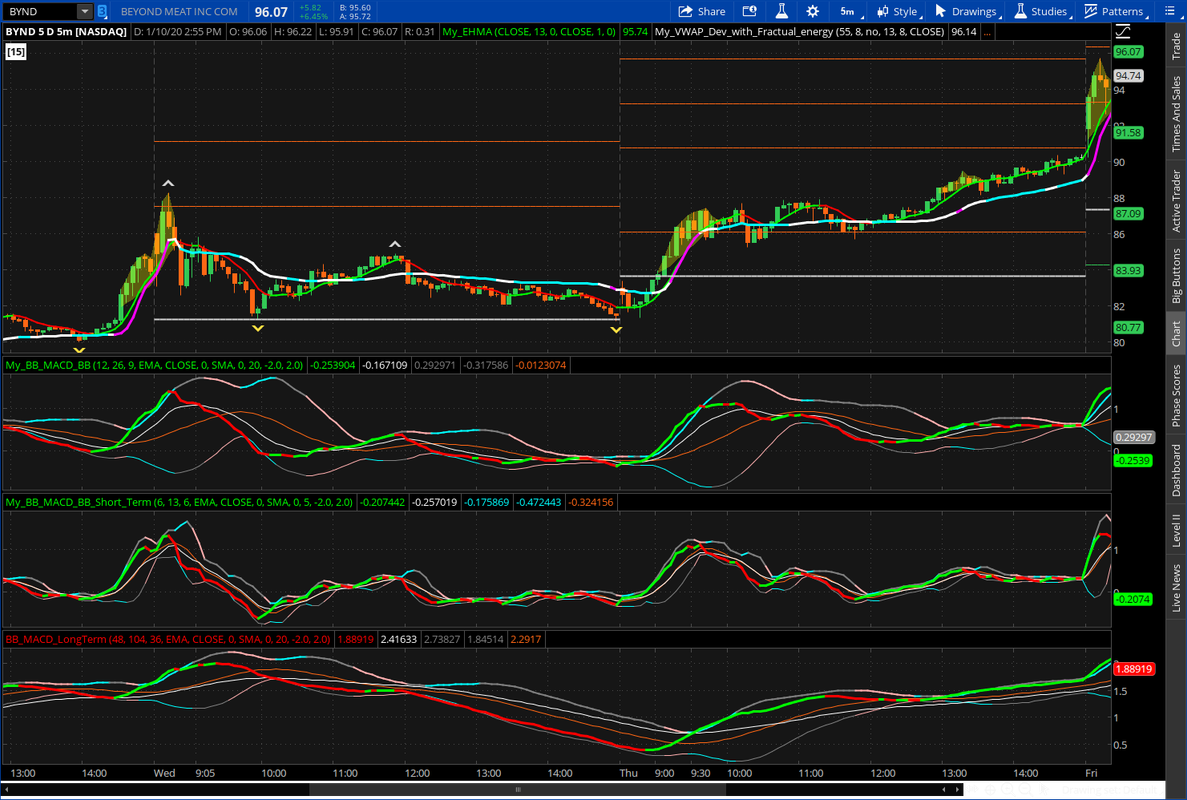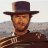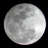# Ultimate MACD Indicator for ThinkorSwim#### horserider

##### Well-known member
VIP
@Lambert58 A screenshot might help to solve the problem.

L

#### Lambert58

##### New member
Ok, I am reading how to post a pic from Imgur.com. Thank you for taking the time to respond, appreciated.

L

#### Lambert58

##### New memberPlease let me know if this can be seen.

I

#### imnobody

##### MemberPlease let me know if this can be seen.
edit study
uncheck data box on bottom portion#### horserider

##### Well-known member
VIP
@Lambert58 You have 2 studies in the same study panel. Many times this will make the scaling do crazy things. If you want to use both of those studies put each in it's own lower panel.

L

#### Lambert58

##### New member
Good Morning, I wanted to say Thank you to both of you, your responses helped me a great deal.#### C-Note Johnson

##### New member
To celebrate the Appreciation Day here is a MACD indicator. It uses a color coded MACD line to show changes. There is a moving average of the MACD signal line. And MACD bands to show volatility. The signal would be the cross of MACD (Green/RED) and signal line (White). A photo is also below to give tips on trading the indicator. The lengths can be adjusted to suit your trading.Here is the code:
Code:
``````# Ultimate MACD by Horserider 8/30/2019

declare lower;
input fastLength = 6;
input slowLength = 13;
input MACDLength = 6;
input AverageTypeMACD = {SMA, default EMA, Wilders};

input price = close;
input displace = 0;

def MACD_Data = MACD(fastLength = fastLength, slowLength = slowLength, MACDLength = MACDLength);
plot MACD_Line = MACD_Data;

MACD_Line.DefineColor("Up", Color.GREEN);
MACD_Line.DefineColor("Down", Color.RED);
MACD_Line.DefineColor("Even", Color.WHITE);
MACD_Line.AssignValueColor(if MACD_Line > MACD_Line then MACD_Line.Color("Up") else (if MACD_Line == MACD_Line then MACD_Line.Color("Even") else MACD_Line.Color("Down")));
MACD_Line.SetLineWeight(3);

def Value;
plot Avg;
switch (AverageTypeMACD) {
case SMA:
Value = Average(price, fastLength) - Average(price, slowLength);
Avg = Average(Value, MACDLength);
case EMA:
Value = ExpAverage(price, fastLength) - ExpAverage(price, slowLength);
Avg = ExpAverage(Value, MACDLength);

case Wilders:
Value = WildersAverage(price, fastLength) - WildersAverage(price, slowLength);
Avg = ExpAverage(Value, MACDLength);
}
Avg.SetDefaultColor(Color.WHITE);

#plot BB;
#Bollinger BandsSMA,EMA
input AverageTypeBB = {default SMA, EMA, HMA};
input displaceBB = 0;
input lengthBB = 20;
input Num_Dev_Dn = -2.0;
input Num_Dev_up = 2.0;

plot upperBand;
plot lowerBand;
def midline;

switch (AverageTypeBB) {
case SMA:
upperBand = reference BollingerBands(MACD_Line, displaceBB, lengthBB, Num_Dev_Dn, Num_Dev_up).UpperBand;
lowerBand = reference BollingerBands(MACD_Line, displaceBB, lengthBB, Num_Dev_Dn, Num_Dev_up).LowerBand;
midline   = reference BollingerBands(MACD_Line, displaceBB, lengthBB, Num_Dev_Dn, Num_Dev_up).Midline;
case EMA:
upperBand = reference BollingerBands(MACD_Line, displaceBB, lengthBB, Num_Dev_Dn, Num_Dev_up, averageType = AverageType.EXPONENTIAL).UpperBand;
lowerBand = reference BollingerBands(MACD_Line, displaceBB, lengthBB, Num_Dev_Dn, Num_Dev_up, averageType = AverageType.EXPONENTIAL).LowerBand;
midline   = reference BollingerBands(MACD_Line, displaceBB, lengthBB, Num_Dev_Dn, Num_Dev_up, averageType = AverageType.EXPONENTIAL).Midline;
case HMA:
upperBand = reference BollingerBands(MACD_Line, displaceBB, lengthBB, Num_Dev_Dn, Num_Dev_up, averageType = AverageType.EXPONENTIAL).UpperBand;
lowerBand = reference BollingerBands(MACD_Line, displaceBB, lengthBB, Num_Dev_Dn, Num_Dev_up, averageType = AverageType.EXPONENTIAL).LowerBand;
midline   = reference BollingerBands(MACD_Line, displaceBB, lengthBB, Num_Dev_Dn, Num_Dev_up, averageType = AverageType.EXPONENTIAL).Midline;
}

upperBand.SetDefaultColor(Color.GRAY);
upperBand.DefineColor("Up", Color.CYAN);
upperBand.DefineColor("Down", Color.PINK);
upperBand.DefineColor("Even", Color.GRAY);
upperBand.AssignValueColor(if upperBand > upperBand and lowerBand < lowerBand then upperBand.Color("Up") else (if upperBand < upperBand and lowerBand > lowerBand then upperBand.Color("Down") else upperBand.Color("Even")));
upperBand.SetLineWeight(2);

lowerBand.SetDefaultColor(Color.GRAY);
lowerBand.DefineColor("Up", Color.CYAN);
lowerBand.DefineColor("Down", Color.PINK);
lowerBand.DefineColor("Even", Color.GRAY);
lowerBand.AssignValueColor(if upperBand > upperBand and lowerBand < lowerBand then upperBand.Color("Up") else (if upperBand < upperBand and lowerBand > lowerBand then upperBand.Color("Down") else upperBand.Color("Even")));

plot midline1 = midline;``````
Hello Horserider, The MACD with Bollinger bands is very intriguing. I can't wait to try it in my ToS. Is it possible for you to create a shareable link ? If not, can you tell me how to get it into my ToS ? Thank you, SFR#### horserider

##### Well-known member
VIP
Updated the original post to show study versatility for different trading styles.

@C-Note Johnson Check first post. Shares placed there.

E

#### emm11

##### New member
@horserider what are those pivot lines that you have on the original post of the thread, would appreciate if you share the code, thanks#### J007RMC

##### Active member
2019 Donor
VIPCode:
``````plot Data = close;declare lower;

input fastLength = 12;
input slowLength = 26;
input MACDLength = 9;

# Four Pole Filter
script g{
input length  = 4;
input price = OHLC4;
def c;
def w;
def beta;
def alpha;
def G;
c = price;
w = (2 * Double.Pi / length);
beta = (1 - Cos(w)) / (Power(1.414, 2.0 / betaDev) - 1 );
alpha = (-beta + Sqrt(beta * beta + 2 * beta));
G = Power(alpha, 4) * c +
4 * (1 – alpha) * G – 6 * Power( 1 - alpha, 2 ) * G +
4 * Power( 1 - alpha, 3 ) * G - Power( 1 - alpha, 4 ) * G;
plot Line = G;
}
# Modified MACD
plot Value = g(length = fastLength) - g(length = slowLength);
plot Avg = g(price = Value, length = MACDLength);
plot Diff = Value - Avg;
plot ZeroLine = 0;

Value.SetDefaultColor(GetColor(1));
Avg.SetDefaultColor(GetColor(8));
Diff.SetDefaultColor(GetColor(5));
Diff.SetPaintingStrategy(PaintingStrategy.HISTOGRAM);
Diff.SetLineWeight(3);
Diff.DefineColor("Positive and Up", Color.GREEN);
Diff.DefineColor("Positive and Down", Color.DARK_GREEN);
Diff.DefineColor("Negative and Down", Color.RED);
Diff.DefineColor("Negative and Up", Color.DARK_RED);
Diff.AssignValueColor(if Diff >= 0 then if Diff > Diff then Diff.color("Positive and Up") else Diff.color("Positive and Down") else if Diff < Diff then Diff.color("Negative and Down") else Diff.color("Negative and Up"));
ZeroLine.SetDefaultColor(GetColor(0));
# End Code Modified MACD - Gaussian``````

Last edited by a moderator:#### horserider

##### Well-known member
VIP
Here is the header for this study.

# MACD with a more Normal Distribution
# Mobius
# V01.09.2015
#Hint: Plots a Gaussian distribution. If Normal Distribution is met, then at minimum, 68.2% of the close values should be inside a One Standard Deviation Envelope and 95.4% of the close values should be inside a 2 Standard Deviation Envelope.#### horserider

##### Well-known member
VIP
•A

#### Ahmar824

##### Member
VIP
Update to show versatility of the study to fit different trading styles. Will include shares to each variation but same can be accomplished by changing the inputs.
Standard MACD 12,26,9 BB length 20. https://tos.mx/sw3SJkx
Short term MACD 6, 13, 6 BB length 5. https://tos.mx/TIERRoZ
Long term MACD 48, 104, 36 BBlength 20. https://tos.mx/wpJy7YKOriginal post is below.

To celebrate the Appreciation Day here is a MACD indicator. It uses a color coded MACD line to show changes. There is a moving average of the MACD signal line. And MACD bands to show volatility. The signal would be the cross of MACD (Green/RED) and signal line (White). A photo is also below to give tips on trading the indicator. The lengths can be adjusted to suit your trading.Here is the code:
Code:
``````# Ultimate MACD by Horserider 8/30/2019

declare lower;
input fastLength = 6;
input slowLength = 13;
input MACDLength = 6;
input AverageTypeMACD = {SMA, default EMA, Wilders};

input price = close;
input displace = 0;

def MACD_Data = MACD(fastLength = fastLength, slowLength = slowLength, MACDLength = MACDLength);
plot MACD_Line = MACD_Data;

MACD_Line.DefineColor("Up", Color.GREEN);
MACD_Line.DefineColor("Down", Color.RED);
MACD_Line.DefineColor("Even", Color.WHITE);
MACD_Line.AssignValueColor(if MACD_Line > MACD_Line then MACD_Line.Color("Up") else (if MACD_Line == MACD_Line then MACD_Line.Color("Even") else MACD_Line.Color("Down")));
MACD_Line.SetLineWeight(3);

def Value;
plot Avg;
switch (AverageTypeMACD) {
case SMA:
Value = Average(price, fastLength) - Average(price, slowLength);
Avg = Average(Value, MACDLength);
case EMA:
Value = ExpAverage(price, fastLength) - ExpAverage(price, slowLength);
Avg = ExpAverage(Value, MACDLength);

case Wilders:
Value = WildersAverage(price, fastLength) - WildersAverage(price, slowLength);
Avg = ExpAverage(Value, MACDLength);
}
Avg.SetDefaultColor(Color.WHITE);

#plot BB;
#Bollinger BandsSMA,EMA
input AverageTypeBB = {default SMA, EMA, HMA};
input displaceBB = 0;
input lengthBB = 20;
input Num_Dev_Dn = -2.0;
input Num_Dev_up = 2.0;

plot upperBand;
plot lowerBand;
def midline;

switch (AverageTypeBB) {
case SMA:
upperBand = reference BollingerBands(MACD_Line, displaceBB, lengthBB, Num_Dev_Dn, Num_Dev_up).UpperBand;
lowerBand = reference BollingerBands(MACD_Line, displaceBB, lengthBB, Num_Dev_Dn, Num_Dev_up).LowerBand;
midline   = reference BollingerBands(MACD_Line, displaceBB, lengthBB, Num_Dev_Dn, Num_Dev_up).Midline;
case EMA:
upperBand = reference BollingerBands(MACD_Line, displaceBB, lengthBB, Num_Dev_Dn, Num_Dev_up, averageType = AverageType.EXPONENTIAL).UpperBand;
lowerBand = reference BollingerBands(MACD_Line, displaceBB, lengthBB, Num_Dev_Dn, Num_Dev_up, averageType = AverageType.EXPONENTIAL).LowerBand;
midline   = reference BollingerBands(MACD_Line, displaceBB, lengthBB, Num_Dev_Dn, Num_Dev_up, averageType = AverageType.EXPONENTIAL).Midline;
case HMA:
upperBand = reference BollingerBands(MACD_Line, displaceBB, lengthBB, Num_Dev_Dn, Num_Dev_up, averageType = AverageType.EXPONENTIAL).UpperBand;
lowerBand = reference BollingerBands(MACD_Line, displaceBB, lengthBB, Num_Dev_Dn, Num_Dev_up, averageType = AverageType.EXPONENTIAL).LowerBand;
midline   = reference BollingerBands(MACD_Line, displaceBB, lengthBB, Num_Dev_Dn, Num_Dev_up, averageType = AverageType.EXPONENTIAL).Midline;
}

upperBand.SetDefaultColor(Color.GRAY);
upperBand.DefineColor("Up", Color.CYAN);
upperBand.DefineColor("Down", Color.PINK);
upperBand.DefineColor("Even", Color.GRAY);
upperBand.AssignValueColor(if upperBand > upperBand and lowerBand < lowerBand then upperBand.Color("Up") else (if upperBand < upperBand and lowerBand > lowerBand then upperBand.Color("Down") else upperBand.Color("Even")));
upperBand.SetLineWeight(2);

lowerBand.SetDefaultColor(Color.GRAY);
lowerBand.DefineColor("Up", Color.CYAN);
lowerBand.DefineColor("Down", Color.PINK);
lowerBand.DefineColor("Even", Color.GRAY);
lowerBand.AssignValueColor(if upperBand > upperBand and lowerBand < lowerBand then upperBand.Color("Up") else (if upperBand < upperBand and lowerBand > lowerBand then upperBand.Color("Down") else upperBand.Color("Even")));

plot midline1 = midline;``````
How do you add it on thinkorswim platform? Is there a video that I can watch?

A

#### Ahmar824

##### Member
VIP
Update to show versatility of the study to fit different trading styles. Will include shares to each variation but same can be accomplished by changing the inputs.
Standard MACD 12,26,9 BB length 20. https://tos.mx/sw3SJkx
Short term MACD 6, 13, 6 BB length 5. https://tos.mx/TIERRoZ
Long term MACD 48, 104, 36 BBlength 20. https://tos.mx/wpJy7YKOriginal post is below.

To celebrate the Appreciation Day here is a MACD indicator. It uses a color coded MACD line to show changes. There is a moving average of the MACD signal line. And MACD bands to show volatility. The signal would be the cross of MACD (Green/RED) and signal line (White). A photo is also below to give tips on trading the indicator. The lengths can be adjusted to suit your trading.Here is the code:
Code:
``````# Ultimate MACD by Horserider 8/30/2019

declare lower;
input fastLength = 6;
input slowLength = 13;
input MACDLength = 6;
input AverageTypeMACD = {SMA, default EMA, Wilders};

input price = close;
input displace = 0;

def MACD_Data = MACD(fastLength = fastLength, slowLength = slowLength, MACDLength = MACDLength);
plot MACD_Line = MACD_Data;

MACD_Line.DefineColor("Up", Color.GREEN);
MACD_Line.DefineColor("Down", Color.RED);
MACD_Line.DefineColor("Even", Color.WHITE);
MACD_Line.AssignValueColor(if MACD_Line > MACD_Line then MACD_Line.Color("Up") else (if MACD_Line == MACD_Line then MACD_Line.Color("Even") else MACD_Line.Color("Down")));
MACD_Line.SetLineWeight(3);

def Value;
plot Avg;
switch (AverageTypeMACD) {
case SMA:
Value = Average(price, fastLength) - Average(price, slowLength);
Avg = Average(Value, MACDLength);
case EMA:
Value = ExpAverage(price, fastLength) - ExpAverage(price, slowLength);
Avg = ExpAverage(Value, MACDLength);

case Wilders:
Value = WildersAverage(price, fastLength) - WildersAverage(price, slowLength);
Avg = ExpAverage(Value, MACDLength);
}
Avg.SetDefaultColor(Color.WHITE);

#plot BB;
#Bollinger BandsSMA,EMA
input AverageTypeBB = {default SMA, EMA, HMA};
input displaceBB = 0;
input lengthBB = 20;
input Num_Dev_Dn = -2.0;
input Num_Dev_up = 2.0;

plot upperBand;
plot lowerBand;
def midline;

switch (AverageTypeBB) {
case SMA:
upperBand = reference BollingerBands(MACD_Line, displaceBB, lengthBB, Num_Dev_Dn, Num_Dev_up).UpperBand;
lowerBand = reference BollingerBands(MACD_Line, displaceBB, lengthBB, Num_Dev_Dn, Num_Dev_up).LowerBand;
midline   = reference BollingerBands(MACD_Line, displaceBB, lengthBB, Num_Dev_Dn, Num_Dev_up).Midline;
case EMA:
upperBand = reference BollingerBands(MACD_Line, displaceBB, lengthBB, Num_Dev_Dn, Num_Dev_up, averageType = AverageType.EXPONENTIAL).UpperBand;
lowerBand = reference BollingerBands(MACD_Line, displaceBB, lengthBB, Num_Dev_Dn, Num_Dev_up, averageType = AverageType.EXPONENTIAL).LowerBand;
midline   = reference BollingerBands(MACD_Line, displaceBB, lengthBB, Num_Dev_Dn, Num_Dev_up, averageType = AverageType.EXPONENTIAL).Midline;
case HMA:
upperBand = reference BollingerBands(MACD_Line, displaceBB, lengthBB, Num_Dev_Dn, Num_Dev_up, averageType = AverageType.EXPONENTIAL).UpperBand;
lowerBand = reference BollingerBands(MACD_Line, displaceBB, lengthBB, Num_Dev_Dn, Num_Dev_up, averageType = AverageType.EXPONENTIAL).LowerBand;
midline   = reference BollingerBands(MACD_Line, displaceBB, lengthBB, Num_Dev_Dn, Num_Dev_up, averageType = AverageType.EXPONENTIAL).Midline;
}

upperBand.SetDefaultColor(Color.GRAY);
upperBand.DefineColor("Up", Color.CYAN);
upperBand.DefineColor("Down", Color.PINK);
upperBand.DefineColor("Even", Color.GRAY);
upperBand.AssignValueColor(if upperBand > upperBand and lowerBand < lowerBand then upperBand.Color("Up") else (if upperBand < upperBand and lowerBand > lowerBand then upperBand.Color("Down") else upperBand.Color("Even")));
upperBand.SetLineWeight(2);

lowerBand.SetDefaultColor(Color.GRAY);
lowerBand.DefineColor("Up", Color.CYAN);
lowerBand.DefineColor("Down", Color.PINK);
lowerBand.DefineColor("Even", Color.GRAY);
lowerBand.AssignValueColor(if upperBand > upperBand and lowerBand < lowerBand then upperBand.Color("Up") else (if upperBand < upperBand and lowerBand > lowerBand then upperBand.Color("Down") else upperBand.Color("Even")));

plot midline1 = midline;``````
never mind I got it thank you! But can you add the 0 line please?#### horserider

##### Well-known member
VIP
@Ahmar824 Good suggestion. Zero line added. Everyone code has been updated to add a zero line to show overall strength or weakness of MACD.
Update to the new code or add this to your study if you want a zero line.

plot zero = 0;
zero.setDefaultColor(color.LIGHT_ORANGE);
zero.setLineWeight (1) ;

•ezrollin and dougn#### horserider

##### Well-known member
VIP
?????? Why was 4 pole gaussian MACD added here.#### BenTen

Staff
VIP
@horserider Since there wasn't a lot of info on it from the original post and since it was related to MACD, I'd thought I added it here as an additional comment so that others can also try it out.#### J007RMC

##### Active member
2019 Donor
VIP
lower studyCode:
``````# Zero Lag MACD With Bollinger Bands
# Mobius
# V01.01.2018 Chat Room Request .0.19.2018

declare lower;

input fastLength = 13;
input slowLength = 34;
input MACDLength = 13;
input showBreakoutSignals = yes;

script EMA{
input length = 10;
input price = close;
def K = 2 / (length + 1);
def lag = (length - 1) / 2;
def ZLEMA = K * (2 * price - price[lag]) + (1 - K) * ZLEMA;
plot data = ZLEMA;
}
plot Value = EMA(length = fastLength) - EMA(length = slowLength);
def Avg = EMA(length = MACDLength, price = value);
plot Diff = Value - Avg;
def SD = StDev(Diff, MACDLength);
plot upper = Diff + SD;
plot lower = Diff - SD;
plot ZeroLine = 0;
Value.SetPaintingStrategy(PaintingStrategy.Line_Vs_Points);
Value.AssignValueColor(if Value > Value
then color.green
else color.red);
Diff.SetStyle(Curve.Short_Dash);
Diff.SetLineWeight(1);
Diff.SetDefaultColor(color.gray);
upper.SetDefaultColor(color.gray);
lower.SetDefaultColor(color.gray);
ZeroLine.SetDefaultColor(GetColor(0));
ZeroLine.HideBubble();
ZeroLine.HideTitle();

# End Code Zero Lag MACD``````

Last edited by a moderator:#### J007RMC

##### Active member
2019 Donor
VIP
thanks I'm the worst at knowing where to put this stuff. the above downloaded through One Note date posted: 04/26/2019

•BenTen#### J007RMC

##### Active member
2019 Donor
VIP
Well not wanting to take more screen time but I beleive this to be a better representation of my above posting thanks.YungTrader's Ultimate Indicator Indicators 670Ultimate VWAP Indicator for ThinkorSwim Indicators 54